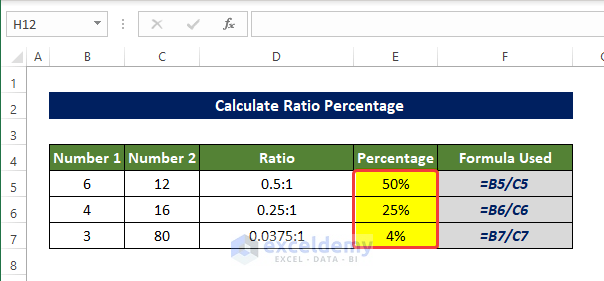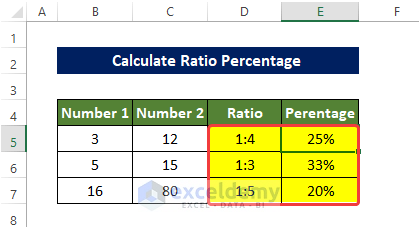# How to Calculate Ratio Percentage in Excel (4 Easy Methods)

Get FREE Advanced Excel Exercises with Solutions!

With ratio, we can visualize the relative size between two or more variables or numbers, In Excel, we also have to deal with ratios a lot. How can we calculate the ratio between two numbers in Excel and determine the percentage after that will discuss here with adequate explanations

## What Is Ratio?

Two or maybe more numbers can be compared by using a ratio to express their relative values.  ratio actually achieves this by division actually. here the number which is going to be divided is called antecedent and the other one is called the consequent.

The expression of ratios generally is a:b, where a and b could be integers decimals or even fractions. Ratio values give us a comparatively easier understanding of the comparison between two variables or numbers. Although they are basically the same thing as division mathematically. But the expression is different.

## 4 Suitable Ways to Calculate Ratio Percentage in Excel

We are going to use the below dataset to demonstrate how we are going to determine the Ratio of Number 1 to Number 2 in the Ratio column and the percentage of Number 1 with respect to the Number 2 column in the Percentage column.

### 1. Using GCD Function

The GCD function will determine the greatest common divisor between the two numbers directly. And then using that number we are going to calculate the ratio between number and percentage thereafter.

Steps

• In the beginning, select the cell D5 and enter the following formula:
`=B5/GCD(B5,C5)&":"&C5/GCD(B5,C5)`
• After entering this formula you will notice that the ratio between cell B5 and C5 is now present in cell D5.• Now drag the Fill Handle to cell D7.
• Then the range of cells D5:D7 is now filled with the ratio of number in the range of cell B5:B7 and range of cells C5:C7.• Now, select the cell E5 and enter the following formula:

`=B5/C5`• Drag the Fill Handle to cell E7, you will see the range of cells E5:E7 now filled with the quotient value of Number 1 column by Number 2 columns.• Now select the range of cells E5 to E7, and from the Home tab, click on the % (Percentage) sign on the Number group.• Then the range of cells E5 to E7 now shows the percentage value of Number 1 of Number 2.This is how we can calculate ratio percentages in Excel using the GCD function.

Breakdown of the Formula

• GCD(B5,C5): This function will return the greatest common divisor between the values in cells B5 and C5
• B5/GCD(B5, C5) and C5/GCD(B5, C5): They will return the quotient of the division of values in cells B5 and C5 by the value returned by the GCD function above.
• B5/GCD(B5,C5)&”:”&C5/GCD(B5,C5): Basically this function will return the values returned above with ratio sign “:”.

### 2. Combining SUBSTITUTE and TEXT Functions

Using the SUBSTITUTE function with the combination of the TEXT function we will calculate the ratio between two numbers and calculate the percentage.

Steps

• In the beginning, select the cell D5 and enter the following formula

`=SUBSTITUTE(TEXT(B5/C5,"######/######"),"/",":")`

• After entering this formula you will notice that the ratio between cell B5 and C5 is now present in cell D5.• Now drag the Fill Handle to cell D7.
• Then the range of cells D5:D7 is now filled with the ratio of number in the range of cells B5:B7 and range of cells C5:C7.• Now select the cell E5 and enter the following formula:
`=B5/C5`• Drag the Fill Handle to cell E7, you will see the range of cells E5:E7 now filled with the quotient value of Number 1 column by Number 2 columns.• Now, select the range of cells E5 to E7. And from the Home tab, click on the percentage sign in the Number group.• After clicking on the percentage sign, now the range of cells E5:E7 is now filled with the percentages of Number 1 with respect to Number 2.This is how we can calculate ratio percentages in Excel using the SUBSTITUTE function.

Breakdown of the Formula

• TEXT(B5/C5,”######/######”): This function will return the quotient of the division of the cell B5 by C5 and format it as fraction.
• SUBSTITUTE(TEXT(B5/C5,”######/######”),”/”,”:”): This formula will substitute the “/” with the “:” in the fraction.

### 3. Applying Simple Division Method

We can simply divide numbers into the first columns by numbers in the second columns to get the ratio. But the output might not be an integer, like other methods.

Steps

• In the beginning, select the cell D5 and enter the following formula
`=(B5/C5)&":"&"1"`
• After entering this formula you will notice that the ratio between cell B5 and C5 is now present in cell D5 with respect to 1.• Now drag the Fill Handle to cell D7.
• Then the range of cells D5:D7 is now filled with the ratio of number in the range of cell B5:B7 and range of cells C5:C7 with respect to 1.• Now select the cell E5 and enter the following formula:
`=B5/C5`• Drag the Fill Handle to cell E7. You will see the range of cells E5:E7 now filled with the quotient value of Number 1 column by Number 2 columns.
• Then select the range of cells D5:D7. And click on the percentage icon from the Home tab in the Numbers group.• After clicking on the percentage icon, now the range of cells E5:E7 is now filled with the percentages of Number 1 with respect to Number 2.

###This is how we can calculate ratio percentages in Excel using simple division.

### 4. Using Combined Formula

The combination of ROUND and TEXT functions will help us to calculate the ratio of two numbers in the Number 1 and Number 2 columns. We also use the LEFT, ROUND, SEARCH, and TEXT functions in this method.

Steps

• In the beginning, select the cell D5 and enter the following formula:

`=LEFT(TEXT(ROUND(B5/C5,5),"###/###"),SEARCH("/",TEXT(ROUND(B5/C5,5),"###/###"))-1)&":"&MID(TEXT(ROUND(B5/C5,5),"###/###"),SEARCH("/",TEXT(ROUND(B5/C5,5),"###/###"))+1,3)`

• After entering this formula you will notice that the ratio between cell B5 and C5 is now present in cell D5.• Now drag the Fill Handle to cell D7.
• Then the range of cells D5:D7 is now filled with the ratio of number in the range of cell B5:B7 and range of cells C5:C7.• In the beginning, select the cell E5 and enter the following formula

`=LEFT(TEXT(ROUND(B5/C5,5),"###/###"),SEARCH("/",TEXT(ROUND(B5/C5,5),"###/###"))-1)/MID(TEXT(ROUND(B5/C5,5),"###/###"),SEARCH("/",TEXT(ROUND(B5/C5,5),"###/###"))+1,3)`

• After entering this formula you will notice that the percentages of cell B5 with respect to C5 are now present in cell E5.• Now drag the Fill Handle to cell E7.
• Now, select the range of cells E5 to E7. And from the Home tab, click on the percentage icon in the Numbers group.• Then the range of cells E5:E7 is now filled with the percentages of the numbers in the range of cells D5:D7. With respect to numbers in the range of cells C5:C7.

###This is how we calculate ratio percentage in Excel by using combined formulas.

Breakdown of the Formula

• ROUND(B5/C5,5): This function will return the quotient of the division of values in cells B5 and C5. And round them up to 5 decimal digits.
• TEXT(ROUND(B5/C5,5),”###/###”): Then this function will format the values as fraction.
• SEARCH(“/”,TEXT(ROUND(B5/C5,5),”###/###”)): This formula will return the location of a particular character inside of a given text. Starting from the left side.
• LEFT(TEXT(ROUND(B5/C5,5),”###/###”),SEARCH(“/”,TEXT(ROUND(B5/C5,5),”###/###”))-1): It will extract the portion of the text from the left side of the text up to a specified location.In this case it will extract up to the location of “/”.
• MID(TEXT(ROUND(B5/C5,5),”###/###”),SEARCH(“/”,TEXT(ROUND(B5/C5,5),”###/###”))+1,3): This formula will extract a specified section length from a particular position. How many characters will be removed is specified. In this case, the three characters after the “/” will be extracted.
• LEFT(TEXT(ROUND(B5/C5,5),”###/###”),SEARCH(“/”,TEXT(ROUND(B5/C5,5),”###/###”))-1)&”:”&MID(TEXT(ROUND(B5/C5,5),”###/###”),SEARCH(“/”,TEXT(ROUND(B5/C5,5),”###/###”))+1,3): This section the extracted part  by LEFT function and MID function is now assembled with keeping “:” in the middle.
• LEFT(TEXT(ROUND(B5/C5,5),”###/###”),SEARCH(“/”,TEXT(ROUND(B5/C5,5),”###/###”))-1)/MID(TEXT(ROUND(B5/C5,5),”###/###”),SEARCH(“/”,TEXT(ROUND(B5/C5,5),”###/###”))+1,3): In this function, the extracted part in LEFT function is now divided by the outcome of the MID function.

## Conclusion

To sum it up, the question “how to calculate Ratio Percentage in Excel’ is answered here in 4 different ways. Starting from using the GCD function. Then using the substitute with TEXT, ROUND, LEFT, MID, SEARCH, ROUND functions, etc, and by simple division. Among all of the methods used here, using the division and the GCD function is comparatively easier to use.

For this problem, a workbook is available for download where you can practice these methods.

Feel free to ask any questions or feedback through the comment section. Any suggestion for the betterment of the Exceldemy community will be highly appreciable.

## Related ArticlesRubayed Razib Suprov

Hi, I am Rubayed Razib Suprov. Graduated from the Bangladesh University of Engineering Technology in Naval Architecture and Marine Engineering department. I joined Exceldemy on March 2022. I have a keen interest in both fluid dynamics and data analysis. I try to teach people what I learned so far about the Excel tool to date, and hope I can make some positive impacts.

1. ReplyYou have an extra 0 in your formula in section 1:
=B5/GCD(B5,C5)&”:”&C5/GCD(B5,C50)

=B5/GCD(B5,C5)&”:”&C5/GCD(B5,C5)

• ReplyRubayed Razib Suprov Dec 26, 2022 at 2:39 PM

Thanks a lot for providing the correction. Both the Image and the Formula has been updated.Advanced Excel Exercises with Solutions PDF